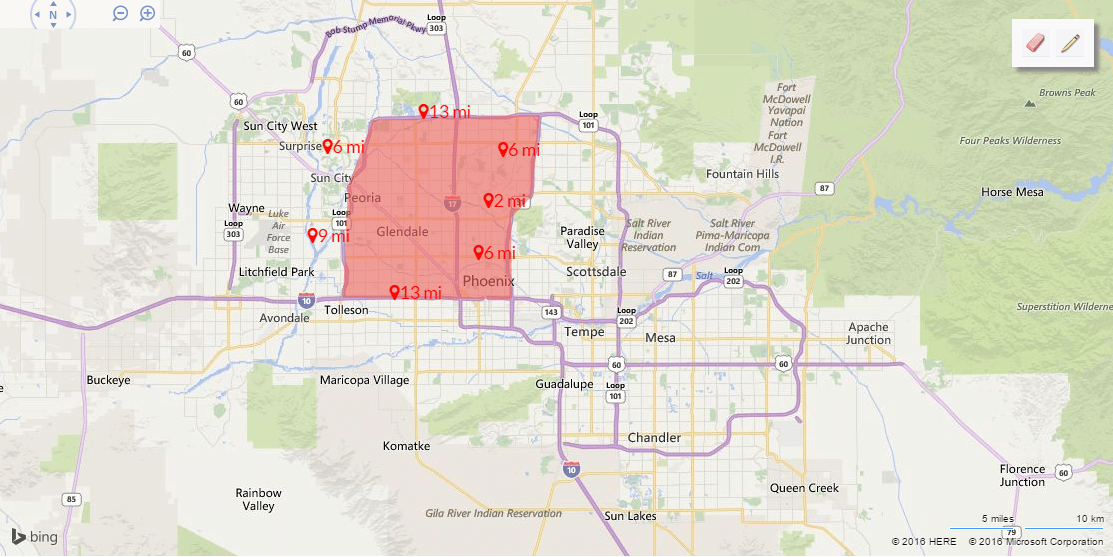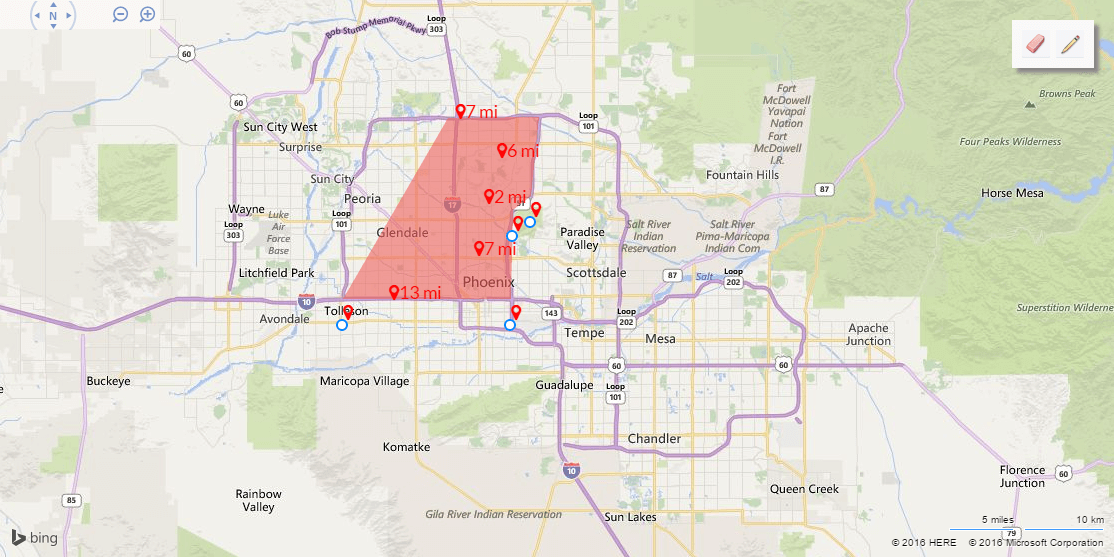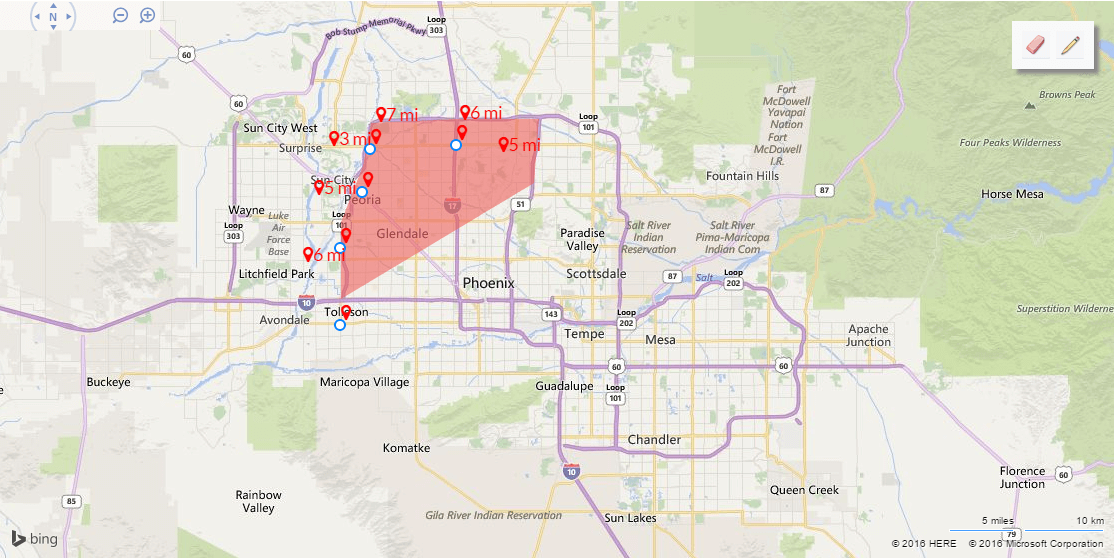The project we're working on needs the ability for a user to define a polygon on a map, like this:Then, we need to save that defined polygon as a SQL Geography type in our database, which we access using Entity Framework. EF supports the DbGeography type, so we are using that to actually send the polygons to the database.

NOTE: for this post, the database server is SQL Server 2008 R2, though I am not sure if the error presented below will occur in other versions of SQL Server.

In order to get to the point where we can create the DbGeography object, though, one of the things we do is submit a string in the well known text format, a commonly-accepted markup language for dealing with vector geometry (i.e. representing areas on a map). We were taking that well-known text and converting it into type DbGeography like so. Our well-known text might look like this:

"{{
"Polygon": "POLYGON((-112.27117 33.4646,-112.27186 33.54476,-112.23959 33.61741,-112.2286 33.666,-111.9972 33.67057,-112.01162 33.5768,-112.03771 33.56307,-112.0384 33.46117,-112.27117 33.4646))"
}}"

Which we then converted into DbGeography by using the FromText() method:

``````var mapObject = DbGeography.FromText(wellKnownText);
``````

This worked for a little while, but then we started noticing a rather strange bug. If we created a polygon by defining the points in a counter-clockwise manner, then that polygon would be created with no issues. A counter-clockwise manner looks like this (first point created is in lower left, near Tolleson):However, if we created a polygon in a clockwise manner, the system would throw a nasty error (first point is again near Tolleson):"The incoming tabular data stream (TDS) remote procedure call (RPC) protocol stream is incorrect. Parameter 2 ("@1"): The supplied value is not a valid instance of data type geography. Check the source data for invalid values. An example of an invalid value is data of numeric type with scale greater than precision."

Which tells me absolutely nothing. It might as well have been written in Klingon.

I took to googling around, and came across a few interesting forum posts, one of which pointed me toward the right answer. The issue was that SQL Geography expects any polygons to be defined using the left-hand rule, which is this:

"If you walk along the polygon from the first point defined to each successive point, the interior of the polygon will be on your left-hand side."

When we are defining the polygons, doing so counter-clockwise places the interior of the polygon as the left-hand side of the imaginary line-walker. However, defining the polygons clockwise makes the exterior of the polygon the line-walker's left-hand side, and so SQL Server thought the polygon we were defining was the entire rest of the world, minus the polygon. Obviously SQL Server won't allow an area so huge (the upper limit for area in SQL Geography is a hemisphere), so it gave us the above error.

So now that we know what the error is (and we care about fixing it), how do we fix it? Like this:

1. First take the well-known text string and create an instance of SQL Geography from it.
2. Then, get the inversion of that object (e.g. get the complete area not "inside" the defined polygon). The two polygons, when combined together, will cover the entire world.
3. Whichever of these two polygons is smaller is the one we want, so use that one.

The code for this looks like the following:

``````public static class DbGeographyExtensions
{
public static DbGeography CreatePolygon(string wellKnownText)
{
//First, get the area defined by the well-known text using left-hand rule
var sqlGeography =
SqlGeography.STGeomFromText(new SqlChars(wellKnownText), DbGeography.DefaultCoordinateSystemId)
.MakeValid();

//Now get the inversion of the above area
var invertedSqlGeography = sqlGeography.ReorientObject();

//Whichever of these is smaller is the enclosed polygon, so we use that one.
if (sqlGeography.STArea() > invertedSqlGeography.STArea())
{
sqlGeography = invertedSqlGeography;
}
return DbSpatialServices.Default.GeographyFromProviderValue(sqlGeography);
}

}
``````

That works like a charm, at least for our uses. Hopefully it'll work for yours as well.

If this helps you out (or you have a better solution, or you can read Klingon), please let me know in the comments!

Happy Coding!